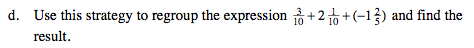### Home > MC2 > Chapter 5 > Lesson 5.1.3 > Problem5-39

5-39.
1. Alex is trying to simplify the expression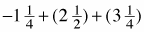. He started by rewriting it like this: Homework Help ✎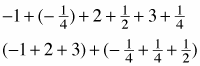1. Why might he have regrouped the expression in this way?

2. Simplify the expression. What is the result?

3. What property was Alex using when he rewrote the problem?

4. Use this strategy to regroup the expression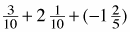and find the result.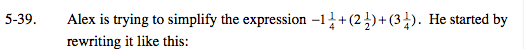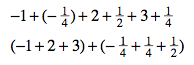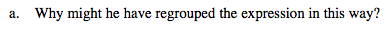What are the differences between the two groups of numbers?

Does it make the equation any easier to solve?

Regrouping simplifies the arithmetic.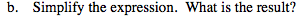First simplify the terms in parentheses.

$4 \frac{1}{2}$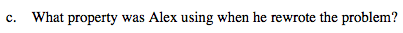What mathematical actions did Alex take?
What property is this?